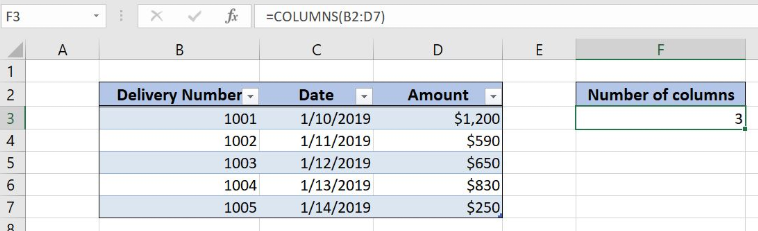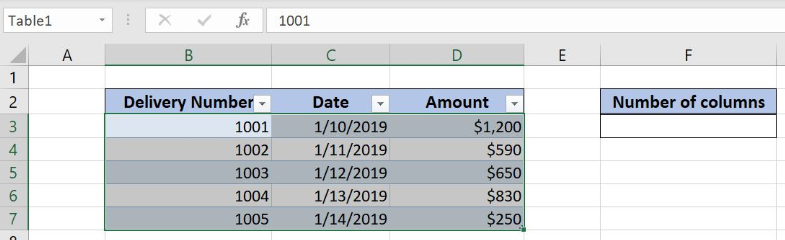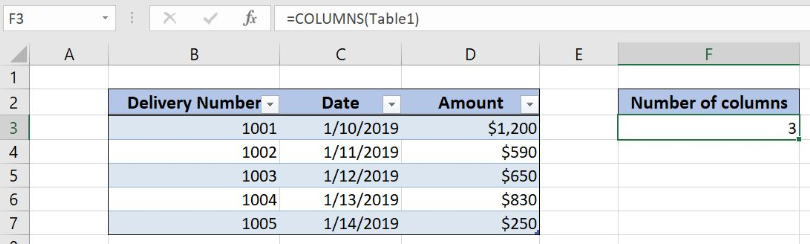Get instant live expert help with Excel or Google Sheets“My Excelchat expert helped me in less than 20 minutes, saving me what would have been 5 hours of work!”

#### Post your problem and you’ll get Expert help in seconds.

Your message must be at least 40 characters
Our professional Expert are available now. Your privacy is guaranteed.

# How to Count Table Columns in Excel

Excel allows a user to count table columns, using the COLUMNS function. This step by step tutorial will assist all levels of Excel users in counting table columns.Figure 1. The result of the formula

## Syntax of the Formula

The generic formula is:

`=COLUMNS(array)`

The parameter of the COLUMNS function is:

• array – an array of cells in a table for which we want to count columns or a table name.

## Setting up Our Data for the Example

Let’s look at the structure of the data we will use. In column B, we have the price and in column C, we have the cost. In column D, we want to calculate a profit margin percentage.Figure 2. Data that we will use in the example

## Counting a Table Columns with a Range

In the first example, we want to count columns in the Excel table, using the table range.

The formula looks like:

`=COLUMNS(B2:D7)`

The parameter array is the range B2:D7.

To apply the formula, we need to follow these steps:

• Select cell F3 and click on it
• Insert the formula: `=COLUMNS(B2:D7)`
• Press enterFigure 3. Counting the table columns with the range

The result in the cell D3 is 3, as there are 3 columns in the table.

## Counting a Table Columns with a Table Name

In this example, we want to count the number of columns in the table, using the table name. The table in the range B2:D7 has name “Table1”.

The formula looks like:

`=COLUMNS(Table1)`

The parameter array is the range Table1.

To apply the formula, we need to follow these steps:

• Select cell F3 and click on it
• Insert the formula: `=COLUMNS(Table1)`
• Press enterFigure 4. Counting the table columns with the table name

As we can see in Figure 4, the result in the cell D3 is 3, the same as in the previous example.

Most of the time, the problem you will need to solve will be more complex than a simple application of a formula or function. If you want to save hours of research and frustration, try our live Excelchat service! Our Excel Experts are available 24/7 to answer any Excel question you may have. We guarantee a connection within 30 seconds and a customized solution within 20 minutes.

### Did this post not answer your question? Get a solution from connecting with the expert.Another blog reader asked this question today on Excelchat:## Subscribe to Excelchat.coAnother blog reader asked this question today on Excelchat: